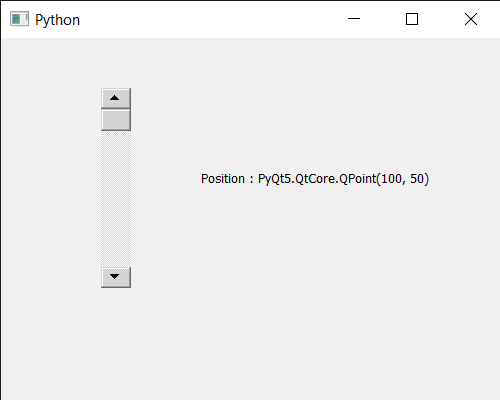GFG App
Open AppBrowser
Continue

# PyQt5 QScrollBar – Getting Its Position in Window

In this article we will see how we can get position of QScrollBar. QScrollBar is a control that enables the user to access parts of a document that is larger than the widget used to display it. Slider is the scroll-able object inside the bar. This property holds the single step. Position is the place where scroll bar is placed in the window. Position can be set with the help of setGeometry method.

In order to do this we will use pos method with the scroll bar object.
Syntax : scroll.pos()
Argument : It takes no argument
Return : It returns QPoint object

Below is the implementation

## Python3

 `# importing libraries` `from` `PyQt5.QtWidgets ``import` `*` `from` `PyQt5 ``import` `QtCore, QtGui` `from` `PyQt5.QtGui ``import` `*` `from` `PyQt5.QtCore ``import` `*` `import` `sys`     `class` `Window(QMainWindow):`   `    ``def` `__init__(``self``):` `        ``super``().__init__()`   `        ``# setting title` `        ``self``.setWindowTitle(``"Python "``)`   `        ``# setting geometry` `        ``self``.setGeometry(``100``, ``100``, ``500``, ``400``)`   `        ``# calling method` `        ``self``.UiComponents()`   `        ``# showing all the widgets` `        ``self``.show()`       `    ``# method for components` `    ``def` `UiComponents(``self``):`   `        ``scroll ``=` `QScrollBar(``self``)`   `        ``# setting geometry of the scroll bar` `        ``scroll.setGeometry(``100``, ``50``, ``30``, ``200``)`   `        ``# making its background color to green` `        ``scroll.setStyleSheet(``"background : lightgrey;"``)`   `        ``# creating a label` `        ``label ``=` `QLabel(``"GeeksforGeeks"``, ``self``)`   `        ``# setting geometry to the label` `        ``label.setGeometry(``200``, ``100``, ``300``, ``80``)`   `        ``# making label multi line` `        ``label.setWordWrap(``True``)`   `        ``# getting value changed signal` `        ``scroll.valueChanged.connect(``lambda``: do_action())`   `        ``# method called when signal is emitted` `        ``def` `do_action():` `            `  `            ``# setting text to the label` `            ``label.setText(``"Current Value : "` `+` `str``(scroll.value()))`     `        ``# getting position of scroll bar` `        ``value ``=` `scroll.pos()`   `        ``# setting text to the label` `        ``label.setText(``"Position : "` `+` `str``(value))`         `# create pyqt5 app` `App ``=` `QApplication(sys.argv)`   `# create the instance of our Window` `window ``=` `Window()`   `# start the app` `sys.exit(App.``exec``())`

Output :My Personal Notes arrow_drop_up